# 傅里叶变换

`${y}_{k+1}=\sum _{j=0}^{n-1}{\omega }^{jk}{x}_{j+1}.$`

$\omega ={e}^{-2\pi i/n}$$n$ 个复单位根之一，其中 $i$ 是虚数单位。对于 $x$$y$，索引 $j$$k$ 的范围为 $0$$n-1$

MATLAB® 中的 `fft` 函数使用快速傅里叶变换算法来计算数据的傅里叶变换。以正弦信号 `x` 为例，该信号是时间 `t` 的函数，频率分量为 15 Hz 和 20 Hz。使用在 10 秒周期内以 $\frac{1}{50}$ 秒为增量进行采样的时间向量。

```Ts = 1/50; t = 0:Ts:10-Ts; x = sin(2*pi*15*t) + sin(2*pi*20*t); plot(t,x) xlabel('Time (seconds)') ylabel('Amplitude')```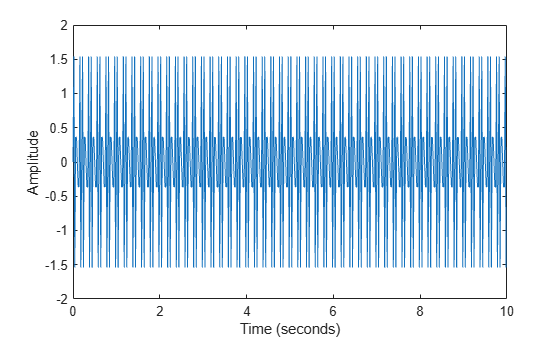```y = fft(x); fs = 1/Ts; f = (0:length(y)-1)*fs/length(y);```

```plot(f,abs(y)) xlabel('Frequency (Hz)') ylabel('Magnitude') title('Magnitude')```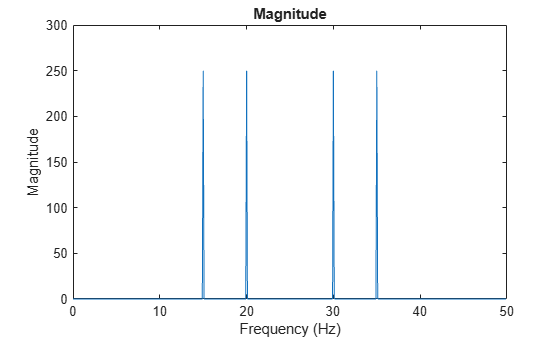```n = length(x); fshift = (-n/2:n/2-1)*(fs/n); yshift = fftshift(y); plot(fshift,abs(yshift)) xlabel('Frequency (Hz)') ylabel('Magnitude')```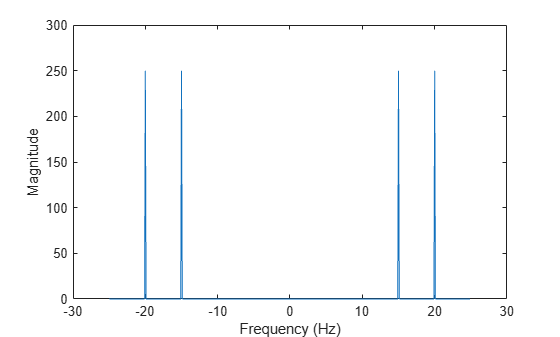### 含噪信号

```rng('default') xnoise = x + 2.5*randn(size(t));```

```ynoise = fft(xnoise); ynoiseshift = fftshift(ynoise); power = abs(ynoiseshift).^2/n; plot(fshift,power) title('Power') xlabel('Frequency (Hz)') ylabel('Power')```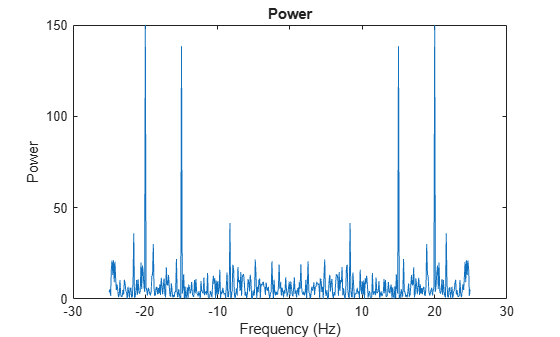### 计算效率

```whaleFile = 'bluewhale.au'; [x,fs] = audioread(whaleFile); whaleMoan = x(2.45e4:3.10e4); t = 10*(0:1/fs:(length(whaleMoan)-1)/fs); plot(t,whaleMoan) xlabel('Time (seconds)') ylabel('Amplitude') xlim([0 t(end)])```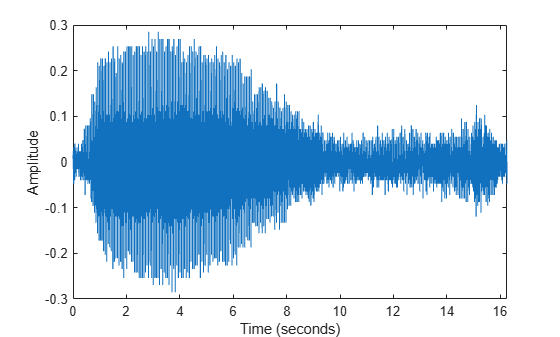```m = length(whaleMoan); n = pow2(nextpow2(m)); y = fft(whaleMoan,n); ```

```f = (0:n-1)*(fs/n)/10; % frequency vector power = abs(y).^2/n; % power spectrum plot(f(1:floor(n/2)),power(1:floor(n/2))) xlabel('Frequency (Hz)') ylabel('Power')```